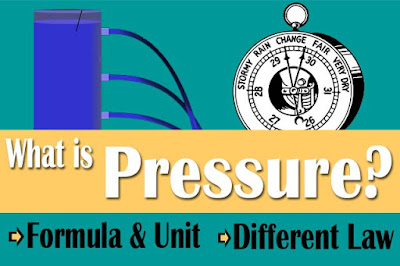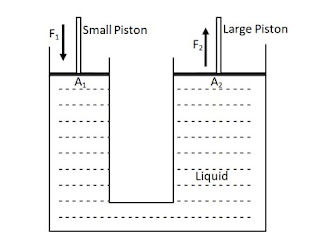# What is pressure? formula and unit | atmospheric pressure

Hello friends, this is the right place for you if you are looking for science related articles and have come this far.

Do you know what is pressurepressure formula and unit, if you don't know? No problems, we teach you in detail.

## What is Pressure?

The amount of force applied per unit area perpendicular is called Pressure. The pressure generated on an object depends on the surface area of ​​that object and the force exerted on that object.

In simple terms, pressure is the effect of a force seen on a surface. The effect of pressure also varies according to the medium. In this article, we will specifically discuss fluid pressure and atmospheric pressure.What is pressure?

### Pressure Formula and Unit

It is denoted by 'P' and the unit of pressure in the SI system is Newton per square meter or Pascal. When 1 Newton force is applied to an area of ​​1 square meter, the resulting pressure is 1 Pascal.

Pressure (P) = F/A  [Where F is the applied force and A is the surface area]

= N (the unit of Force)/ m2(the unit of area)

So, the unit of pressure is N/m2 or, Pascal (Pascal is the Scientist's name)

There are various examples in our daily life which are based on the principle of fluid pressure. For example, lifting large loads with the help of hydraulic machines, in large houses reach the upper floors easily with the help of a lift etc.

### Pascal’s law

The law provided by Pascal in relation to the principle of fluid pressure is called Pascal's law. Pascal full name be Blaise Pascal. He was born in 1623 in France. He was a famous mathematician and philosopher of that time.

"If the pressure is applied to the liquid in a closed vessel at one place, the same pressure is transmitted perpendicularly everywhere."

#### The following Activities can be done to prove this rule.

Activities 1

Take a plastic bag or balloon as shown in the picture, fill it with water and Tie up its mouth tightly. With the help of a needle, make small holes around it and gently squeeze using force from the mouth of the bag. Is the water flowing evenly from every hole? The pressure on the plastic bag increases. Since pressure is transmitted in equal amounts everywhere, water comes out evenly from all the holes.

This experiment proves that by increasing the pressure on one side of the liquid in the closed vessel, the same pressure is transmitted perpendicular everywhere.

### Devices based on Pascal's theory

#### Hydraulic press

The hydraulic press is a device based on Pascal's law. It consists of a small and another large cross sectional area of ​​pipe connected by another horizontal tube. The tube is filled with oil or water. Pressing the big piston brings up the small piston and pressing the small piston lifts the big piston.Hydraulic press

With the help of this, a small weight is applied to a small piston and a large weight is lifted from a large piston.

Example,

Suppose the area of a large tube is 100 meters square and the area of a small tube is 40 meters square. Both have matching pistons. The tube is filled with fluid. Now how much weight does a big piston lift when applying 25 Newton force to a small piston?

Here,

A1 = 40m2

A2 = 100m2

F1 = 25N

F2 = ?

According to Pascal's law, the pressure exerted in the small piston is equal to the pressure exerted in the larger piston.

Or,         P1 = P2

Or,         F1/A1  = F2/A2

25/40 = F2/100

F2 = (25x100)/40

Or,         F2 =  62.5N

So, here it is seen that a big piston can lift a large weight by applying small force to a small piston.

### Density of liquid and up thrust

The mass of liquid per unit area is called the density of liquid. The resultant force of a liquid pushing an object upwards is called up thrust. It is also called upward pressure.

The up thrust on an object fluctuates according to the density of the liquid. That is, if the liquid density is high, then the up thrust in an object is also high.

For example, an egg cannot float in clean water but when we add some salt to the water the water density is high and the egg can float easily.

### Archimedes's Principle

"When we place an object partially or completely in a liquid, the weight of the displaced liquid is equal to the up thrust."

This is called the Archimedes's Principle. Archimedes was a mathematician and physicist born in 287 BC in the city of Sicily in Italy. The principle applies to both liquid and gas.

Activities 2

Take a eureka can, pan balance, spring balance, beaker and a small stone attached with thread. Keep the weight in spring balance. Fill water up to the tube in Eureka. Fit the beaker on the pan balance as shown in the figure.

After this arrangement, add stone to Eureka water with the help of thread and spring balance. Measure the weight of the beaker and the displaced water with the help of pan balance and also measure the weight of stone in air.Archimedes's Principle

Suppose,

Weight of stone in air = W1

Weight of beaker = W2

Weight of stone in water = W3

Weight of displaced water with Beaker = W4

Weight lost by object in water = W1- W3

Up thrust of Water = W1- W3

Weight lost by object in water = Weight of displaced water with Beaker - Weight of beaker

\ W1- W3 = W4-W2

\ Up thrust = Weight of displaced liquid

### Law of floatation

"An object that floats on a liquid displaces a fluid equal to its own." This is called the law of floatation.

According to this law, all objects placed in liquid displace the weight of water equal to their weight for floating.

Large ships made of iron float on water even after much loading, but an iron fort is sunk. This means that density alone does not affect the floating of an object in a liquid. For floating an object in a liquid, its density as well as its size also affects it.

### What is Atmospheric Pressure?

The layer of air (mixture of different gases) around the earth is called atmosphere. It is a mixture of various gases, water vapor, smoke, washes, which are spread over about 1000 km above the earth.

Due to the weight of air in the atmosphere, it exerts pressure on the earth's surface, which is called atmospheric pressure.

"Atmospheric pressure is the pressure of air(mixture of different gases) in the unit area of the Earth's surface"

The Atmospheric pressure at sea level is 106N/m2 or 760mm Hg, which is called standard atmospheric pressure.

#### Devices based on atmospheric pressure

Mercury Barometer : Mercury barometer is a device based on atmospheric pressure which is used for measuring atmospheric pressure.

Syringe : Syringe is an instrument based on atmospheric pressure which is used for treatment purpose.

Air Pump : Air pump is an instrument based on atmospheric pressure which is used for filling air in different object like tube of bicycle, motorcycle, balloon, football etc.

In the end

In this post I have told you what is pressurepressure formula and unit, different law related with pressure, different device and atmospheric pressure etc. I hope you have read this article completely and you will know detail about these topics.

If you don't understand any topics, then you can comment below, and if you like this post, then surely you will share this post with your friends. I hope you will come to our site daily for new information. Thanks a lot.